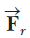# Problem: The potential energy of a system of two particles separated by a distance r is given by U(r) = A/r, where A is a constant. Find the radial force  that each particle exerts on the other.

###### FREE Expert Solution

In this problem, we are required to apply the relationship between potential energy and force.

Force:

79% (183 ratings)###### Problem Details

The potential energy of a system of two particles separated by a distance r is given by U(r) = A/r, where is a constant. Find the radial forcethat each particle exerts on the other.